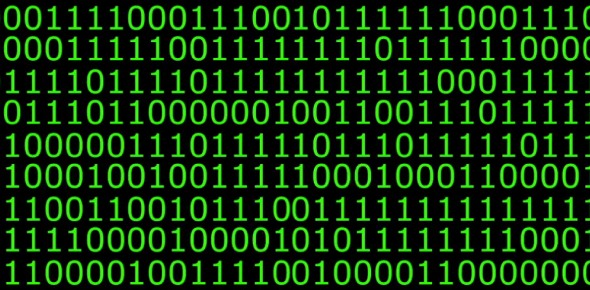# Number Representation In Binary, Decimal And Hexadecimal

9 Questions | Total Attempts: 145SettingsMathematics at times is presented in ways, which seem so hard, confusing and difficult. Among the so thought confusing issues are binary, decimal and hexadecimal concepts. Well, lets see how much you know. Good luck!

• 1.
• 2.
What does the hexadecimal number 2B16 represent in decimal?
• 3.
What is the twos complement value 111011002 in decimal.
• 4.
Represent the number 15.75 in 8 bits fixed point. The decimal point is between the first four and second four binary digits. The number is in pure binary not twos complement.
• 5.
What is the biggest positive number that can be represented in 8 bits two's complement?Answer in decimal NOT binary
• 6.
Represent the number -50 in 8 bits twos complement.
• 7.
Represent the binary value 1111101011001110 as hexadecimal.
• 8.
Which calculation best describes the number of bit patterns that it is possible to store in n bits.
• A.

10n

• B.

2n

• C.

2n-1

• D.

10n-1

Related TopicsBack to top